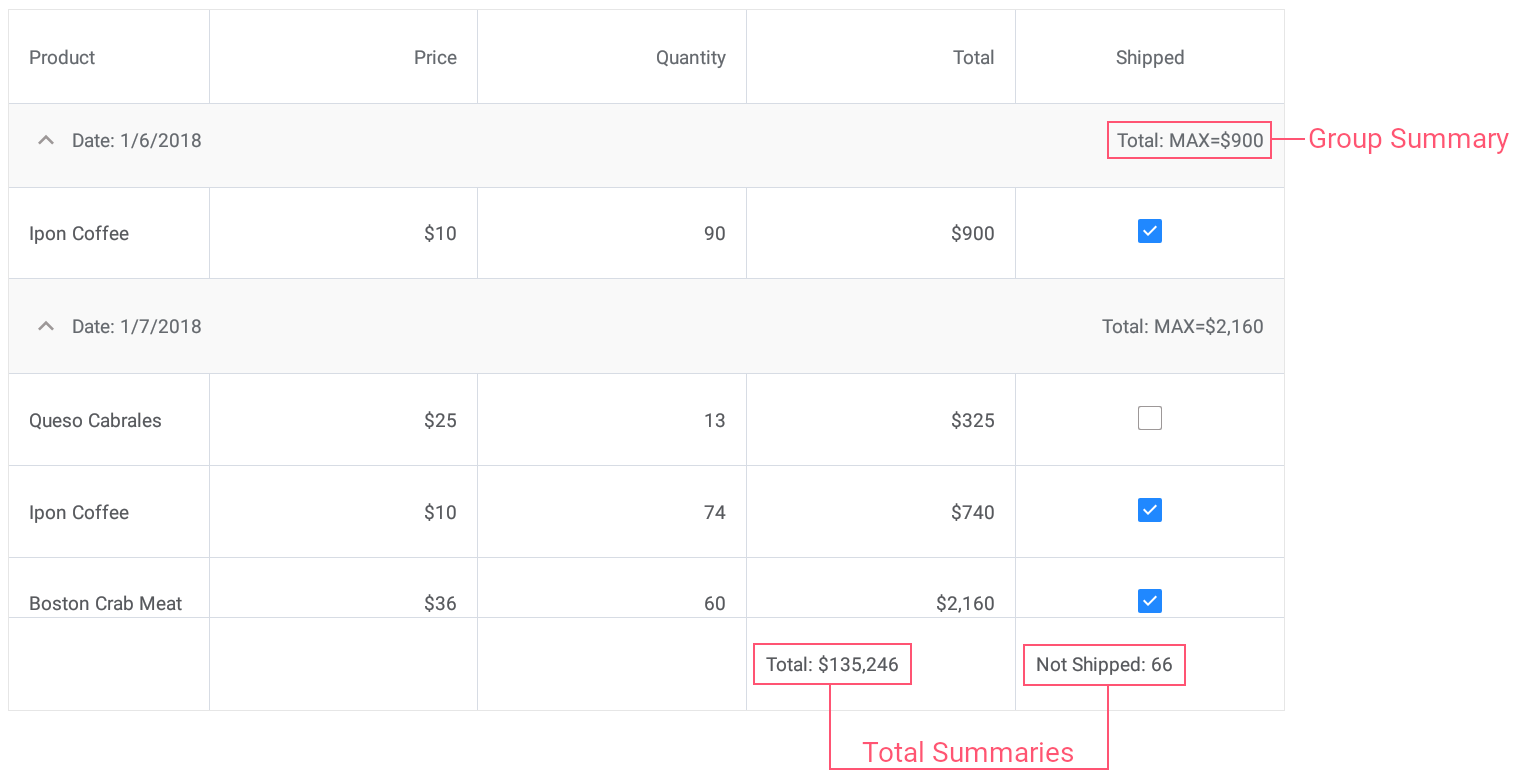21.1
20.2
20.1
19.2
19.1
1.1
The page you are viewing does not exist in version 1.1. This link will take you to the root page.

# CustomSummaryEventArgs.IsTotalSummary Property

Gets whether a total summary value is being calculated.

Namespace: DevExpress.XamarinForms.DataGrid

Assembly: DevExpress.XamarinForms.Grid.dll

## Declaration

``public bool IsTotalSummary { get; }``

## Property Value

Type Description
Boolean

true, if a total summary value is being calculated; otherwise, false.

## Example

This example demonstrates how to use predefined aggregate functions and custom rule to calculate group and total summaries for grid columns.• Set the group summary to display the maximum Total value for each group of records.
• Set the total summary to calculate the sum of values in the Total column.
• Set the custom total summary to count the number of orders whose value in the Shipped column is false (to count orders that are not shipped).
``````<dxg:DataGridView x:Name="grid" ItemsSource="{Binding Orders}"
CalculateCustomSummary="OnCalculateCustomSummary">
<!-- ... -->
<dxg:DataGridView.GroupSummaries>
<dxg:GridColumnSummary FieldName="Total" Type="Max"/>
</dxg:DataGridView.GroupSummaries>

<dxg:DataGridView.TotalSummaries>
<dxg:GridColumnSummary FieldName="Total" Type="Sum"
DisplayFormat="Total: {0:C0}"/>
<dxg:GridColumnSummary FieldName="Shipped" Type="Custom"
DisplayFormat="Not Shipped: {0}"/>
</dxg:DataGridView.TotalSummaries>
</dxg:DataGridView>
``````
``````int count;
// ...

void OnCalculateCustomSummary(object sender, CustomSummaryEventArgs e) {
if (e.FieldName.ToString () == "Shipped")
if (e.IsTotalSummary){
if (e.SummaryProcess == CustomSummaryProcess.Start) {
count = 0;
}
if (e.SummaryProcess == CustomSummaryProcess.Calculate) {
if (!(bool)e.FieldValue)
count++;
e.TotalValue = count;
}
}
}
``````
See Also